# 基于视觉伺服的工业机器人系统研究（摄像机标定、手眼标定、目标单目定位）

## 一、标定技术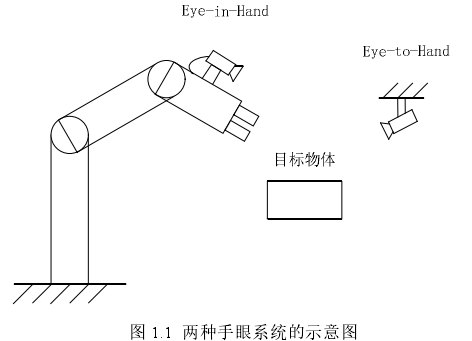### 1、摄像机标定技术

（1）理论部分：

1）得到图片像素坐标系P与摄像机坐标系C之间的转换矩阵T1，准确说应该是摄像机坐标系转化为图片像素坐标系的转换矩阵。可表示为：

P=T1*C;

2）得到摄像相机坐标系C与棋盘上建立的世界坐标系G之间的转换矩阵T2，准确说应该是坐标系G转化为摄像机坐标系的转换矩阵。可表示为：

C=T2*G;

（2）方法：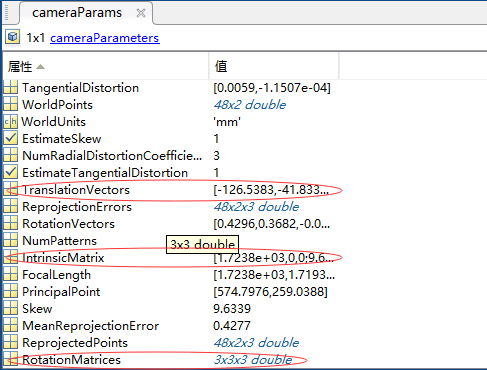### 2、手眼标定技术

（1）理论部分：

C=T3*H;

C是两个摄像机坐标系之间的变换矩阵。可以根据上述任一两张标定图片所得的两个摄像机标定外参A、B按公式C=A*inv(B)计算得到的。假设上述摄像机标定中有3张标定图片的外参标定结果分别是T21、T22、T23，那么可以得到两个C矩阵：

C1=T21*inv(T22);

C2=T22*inv(T23);

D的求法如下：

D是两个机器手坐标系之间的变换矩阵。假设上述摄像机标定中的3张标定图片所一一对应的机器手坐标系在基坐标系（也可以是工件坐标系或者其他固定的参考坐标系）中的描述矩阵结果分别是H1、H2、H3（H需要从机器人控制器或示教器中读取），那么可以得到两个D矩阵：

D1=inv(H1)*H2;

D2=inv(H2)*H3;

（2）方法：

1）根据摄像机标定已知摄像机外参矩阵T21、T22、T23，还要从机器人控制器中读取T21、T22、T23分别对应的机器手(或工具)坐标系H1、H2、H3。控制器中的坐标系描述矩阵不是直接读取的，它是以平移向量和欧拉角（或四元数）模式存在的，如下：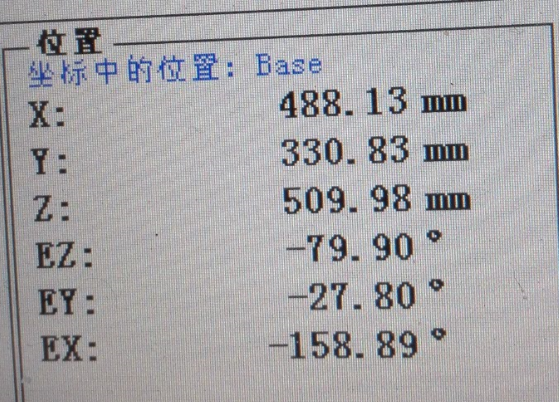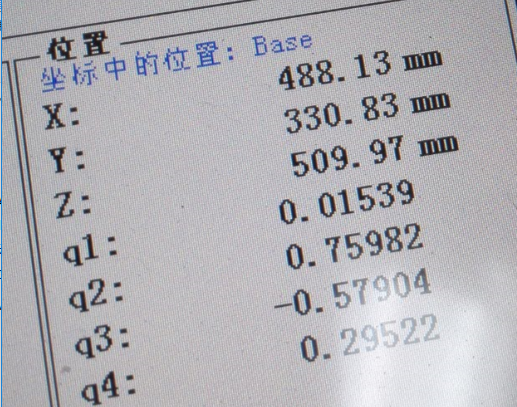C_ext=cat(3, C_ext1, C_ext2, C_ext3);

H=cat(3, H1, H2 ,H3)

function Tch = GetCamera2HandMatrix(C_ext,H)
%   以下变量：
%   C_ext是3个位置的摄像机外参矩阵：3x4x4
%   H1、H2、H3分别是3个位置的机械手坐标系的姿态矩阵：3x4x4
%   Tcg--机器手坐标系（或工具坐标系）在摄像机坐标系中的姿态和位置变换矩阵

%   C1、D1、C2、D2、R、w、q、kc1、kc2、kc3、kd1、kd2、kd3、a、b、c、d、h、y均为临时变量

C1=C_ext(:,:,1)*inv(C_ext(:,:,2))
C2=C_ext(:,:,2)*inv(C_ext(:,:,3))
D1=inv(H(:,:,1))*H(:,:,2)
D2=inv(H(:,:,2))*H(:,:,3)

R=C1(1:3,1:3);
q=acos((trace(R)-1)/2);
w(1,1)=q/(2*sin(q))*(R(3,2)-R(2,3));
w(2,1)=q/(2*sin(q))*(R(1,3)-R(3,1));
w(3,1)=q/(2*sin(q))*(R(2,1)-R(1,2));
kc1=w;

R=C2(1:3,1:3);
q=acos((trace(R)-1)/2);
w(1,1)=q/(2*sin(q))*(R(3,2)-R(2,3));
w(2,1)=q/(2*sin(q))*(R(1,3)-R(3,1));
w(3,1)=q/(2*sin(q))*(R(2,1)-R(1,2));
kc2=w;

R=D1(1:3,1:3);
q=acos((trace(R)-1)/2);
w(1,1)=q/(2*sin(q))*(R(3,2)-R(2,3));
w(2,1)=q/(2*sin(q))*(R(1,3)-R(3,1));
w(3,1)=q/(2*sin(q))*(R(2,1)-R(1,2));
kd1=w;

R=D2(1:3,1:3);
q=acos((trace(R)-1)/2);
w(1,1)=q/(2*sin(q))*(R(3,2)-R(2,3));
w(2,1)=q/(2*sin(q))*(R(1,3)-R(3,1));
w(3,1)=q/(2*sin(q))*(R(2,1)-R(1,2));
kd2=w;

kc3=cross(kc1,kc2);
kd3=cross(kd1,kd2);
a=[kc1 kc2 kc3];
b=[kd1 kd2 kd3];
R=a*inv(b);  %得到旋转关系矩阵

tc1=C1(1:3,4);
tc2=C2(1:3,4);
td1=D1(1:3,4);
td2=D2(1:3,4);
c=R*td1-tc1;
d=R*td2-tc2;
a=C1(1:3,1:3)-[1 0 0;0 1 0;0 0 1];
b=C2(1:3,1:3)-[1 0 0;0 1 0;0 0 1];
h=[a;b];
y=[c;d];
t=inv(h'*h)*h'*y;   %得到平移关系矩阵

Tch=[R t;0 0 0 1];   %得到最终结果
end

### 3、根据标定结果对固定高度目标实现单目定位

（1）理论部分：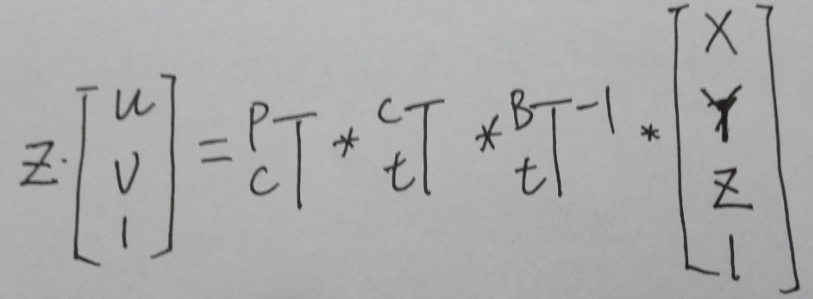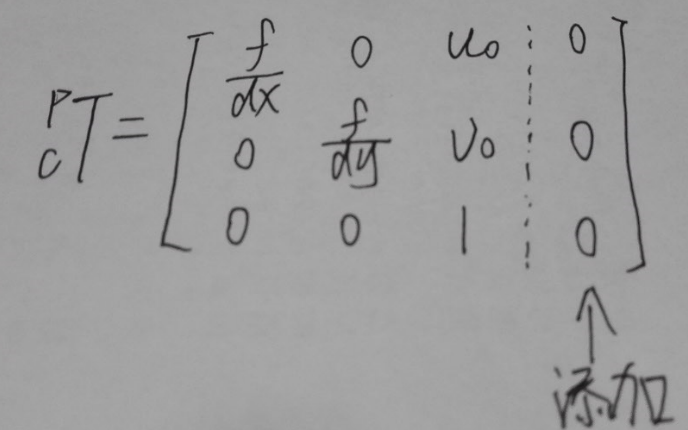（2）代码实现：

function  P= GetObjectLocation( u,v,Gtb)
%   参数(u,v)为目标在图片中的像素坐标
%   参数Gtb是工具在机器人基坐标中的描述矩阵（也就是工具坐标系->基坐标系的变换矩阵）

%内参矩阵
Kl=[ 1851    9.7    550.5  0;
0    1844.4    299.7  0;
0         0    1.0    0];
%摄像机与工具关系矩阵
Gctl= [-0.9620   -0.2974    0.0156   -2.6405;
0.3266   -0.9552    0.0056   59.7141;
0.0130    0.0003    1.0161  145.3381;
0         0         0    1.0000];

G=inv(Gtb);
z=10;  %指定物体的高度
M=Kl*Gctl*G;
Ml=[u*M(3,1)-M(1,1)  u*M(3,2)-M(1,2)  ;   v*M(3,1)-M(2,1)  v*M(3,2)-M(2,2)];
Mr=[M(1,4)-u*M(3,4)-(u*M(3,3)-M(1,3))*z;  M(2,4)-v*M(3,4)-(v*M(3,3)-M(2,3))*z];
P=inv(Ml)*Mr;  %得到物体的位置
end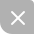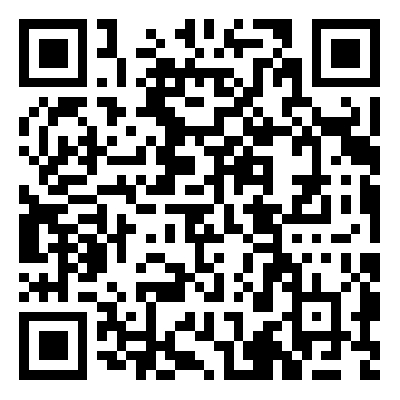UP更新不错过~
05-014244
04-1570
10-04992
11-043518
11-21919
09-152619
11-131499
02-271724
12-039467
11-087297
11-09
03-28
02-05303
08-245万+
03-311万+

### “相关推荐”对你有帮助么？

•非常没帮助
•没帮助
•一般
•有帮助
•非常有帮助被折叠的  条评论 为什么被折叠?到【灌水乐园】发言¥2 ¥4 ¥6 ¥10 ¥20余额支付 (余额：-- )扫码支付获取中扫码支付点击重新获取扫码支付1.余额是钱包充值的虚拟货币，按照1:1的比例进行支付金额的抵扣。
2.余额无法直接购买下载，可以购买VIP、C币套餐、付费专栏及课程。余额充值